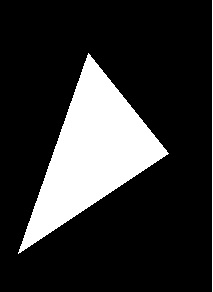# fillpoly() function in C

## Concept

Now the header file graphics.h contains fillpoly() function which is implemented to draw and fill a polygon such as triangle, rectangle, pentagon, hexagon etc. So this function require same arguments as drawpoly().

## Syntax

void fillpoly( int number, int *polypoints );

In this case,number indicates (n + 1) number of points where, n is the number of vertices in a polygon and polypoints points to a sequence of (n*2) integers.

Input

arr[] = {320, 150, 400, 250, 250, 350, 320, 150};

OutputExplanation

So the declaration of fillpoly() contains two arguments: number specifies (n + 1) number of points where n is indicated as the number of vertices in a polygon.The second argument, such as, polypoints points to a sequence of (n * 2) integers. As a result of this,each pair of integers provides x and y coordinates of a point on the polygon. So we indicate (n + 1) points because first point coordinates should be equal to (n + 1)th for drawing a complete figure.

## Example

// C Implementation for fillpoly()
#include <graphics.h>
// driver code
intmain(){
// Here gm1 is Graphics mode which is a computer display mode that
// produces image using pixels. DETECT is a macro defined in
intgd1 = DETECT, gm1;
// Different coordinates for polygon
intarr1[] = {320, 150, 400, 250, 250, 350, 320, 150};
// Here initgraph initializes the
// graphics driver from disk
initgraph(&gd1, &gm1, "");
// fillpoly function
fillpoly(4, arr1);
getch();
// Here closegraph function closes the
// graphics mode and deallocates
// all memory allocated by
// graphics system .
closegraph();
return0;
}

## Output